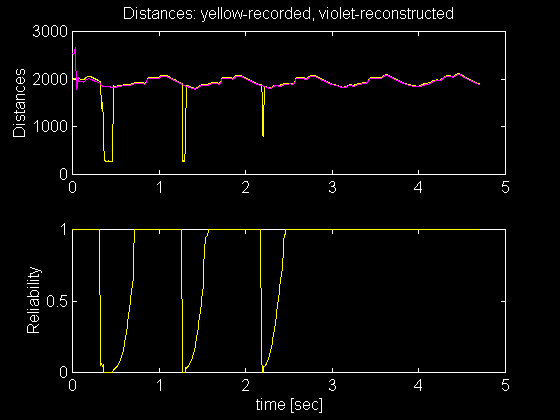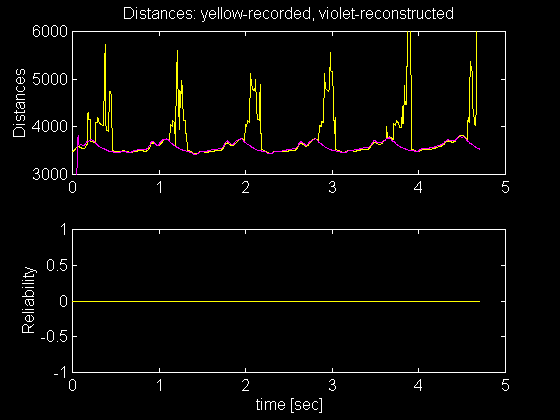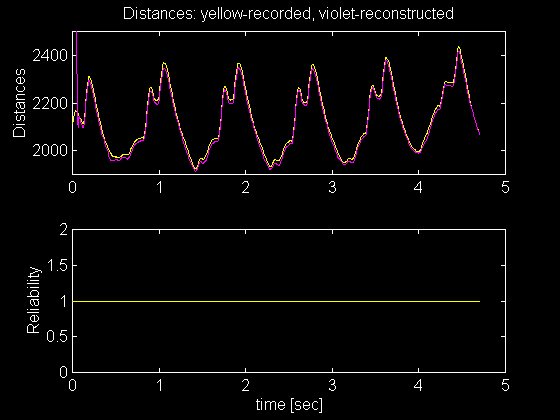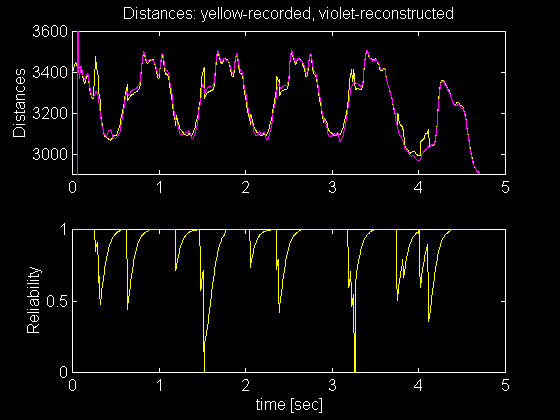Beating Heart Site Map:
Wire-mesh animation | Java source |
Data acquisition | Graphs | Matlab source |
B-spline modelling: [ PowerPoint | HTML ] | Matlab source |
B-spline animation | Java source |

## Graphs of the Measured and Calculated Distances

The following graphs are generated in MATLAB. Input dataset contains distances between sensors mounted on a beating heart, changing over time. For each sampled point in time, distances between 12 sensors are measured. That gives a 12x12 matrix. The value in the matrix at index (i,j) represents the distance between i-th and j-th sensor.

Using measured distances, the positions of all sensors are calculated.

Figures below show distances between only ONE pair of sensors, changing over time. The upper portion of the figures below shows recorded signal (in yellow) and the distances obtained from calculated cartesian coordinates (violet). The lower part shows reliability or "goodnes" of the recorded signal. It has values form zero, for "bad" signals (discontinuities) and one for the signal that appears to be proper (continuous and inside reasonable limits). This value increases over time if the signal remains smooth after a discontinuity.The measured and calculated values differ significantely at times where measured distances rapidly change. That usually occurs due to the hardware measurement error. Even if most of the datapoints for one pair of sensors is bad, correct distances can be recalculated from remaining sensors. One such case is shown in the figure below.If the signal does not have any sudden changes, the "reliability" is considered to be "good", taking the value of 1.Depending on the its "reliability" value, ONE distance between two sensors will have diffenet contribution to the final result of position calculation for ALL sensors.